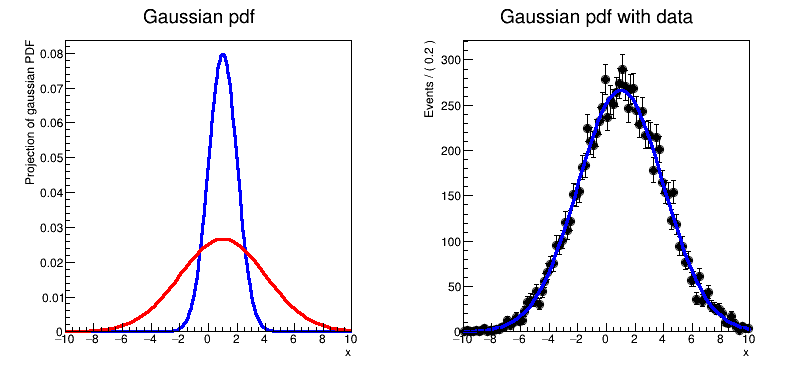# Rf 1 0 1_Basics¶

This tutorial illustrates the basic features of RooFit.

Author: Clemens Lange, Wouter Verkerke (C++ version)
This notebook tutorial was automatically generated with ROOTBOOK-izer from the macro found in the ROOT repository on Saturday, November 28, 2020 at 10:34 AM.

In :
import ROOT

Welcome to JupyROOT 6.23/01


## Set up model¶

Declare variables x,mean,sigma with associated name, title, initial value and allowed range

In :
x = ROOT.RooRealVar("x", "x", -10, 10)
mean = ROOT.RooRealVar("mean", "mean of gaussian", 1, -10, 10)
sigma = ROOT.RooRealVar("sigma", "width of gaussian", 1, 0.1, 10)

RooFit v3.60 -- Developed by Wouter Verkerke and David Kirkby
Copyright (C) 2000-2013 NIKHEF, University of California & Stanford University



Build gaussian pdf in terms of x,mean and sigma

In :
gauss = ROOT.RooGaussian("gauss", "gaussian PDF", x, mean, sigma)


Construct plot frame in 'x'

In :
xframe = x.frame(ROOT.RooFit.Title("Gaussian pdf"))  # RooPlot


## Plot model and change parameter values¶

Plot gauss in frame (i.e. in x)

In :
gauss.plotOn(xframe)

Out:
<cppyy.gbl.RooPlot object at 0x669b4e0>

Change the value of sigma to 3

In :
sigma.setVal(3)


Plot gauss in frame (i.e. in x) and draw frame on canvas

In :
gauss.plotOn(xframe, ROOT.RooFit.LineColor(ROOT.kRed))

Out:
<cppyy.gbl.RooPlot object at 0x669b4e0>

## Generate events¶

Generate a dataset of 1000 events in x from gauss

In :
data = gauss.generate(ROOT.RooArgSet(x), 10000)  # ROOT.RooDataSet


Make a second plot frame in x and draw both the data and the pdf in the frame

In :
xframe2 = x.frame(ROOT.RooFit.Title(
"Gaussian pdf with data"))  # RooPlot
data.plotOn(xframe2)
gauss.plotOn(xframe2)

Out:
<cppyy.gbl.RooPlot object at 0x6b49bf0>

## Fit model to data¶

Fit pdf to data

In :
gauss.fitTo(data)

Out:
<cppyy.gbl.RooFitResult object at 0x(nil)>
[#1] INFO:Minization -- RooMinimizer::optimizeConst: activating const optimization
**********
**    1 **SET PRINT           1
**********
**********
**********
PARAMETER DEFINITIONS:
NO.   NAME         VALUE      STEP SIZE      LIMITS
1 mean         1.00000e+00  2.00000e+00   -1.00000e+01  1.00000e+01
2 sigma        3.00000e+00  9.90000e-01    1.00000e-01  1.00000e+01
**********
**    3 **SET ERR         0.5
**********
**********
**    4 **SET PRINT           1
**********
**********
**    5 **SET STR           1
**********
NOW USING STRATEGY  1: TRY TO BALANCE SPEED AGAINST RELIABILITY
**********
**********
FIRST CALL TO USER FUNCTION AT NEW START POINT, WITH IFLAG=4.
START MIGRAD MINIMIZATION.  STRATEGY  1.  CONVERGENCE WHEN EDM .LT. 1.00e-03
FCN=25019.2 FROM MIGRAD    STATUS=INITIATE       10 CALLS          11 TOTAL
EDM= unknown      STRATEGY= 1      NO ERROR MATRIX
EXT PARAMETER               CURRENT GUESS       STEP         FIRST
NO.   NAME      VALUE            ERROR          SIZE      DERIVATIVE
1  mean         1.00000e+00   2.00000e+00   2.02430e-01  -1.99004e+02
2  sigma        3.00000e+00   9.90000e-01   2.22742e-01   1.98823e+02
ERR DEF= 0.5
MIGRAD WILL VERIFY CONVERGENCE AND ERROR MATRIX.
COVARIANCE MATRIX CALCULATED SUCCESSFULLY
FCN=25018.5 FROM MIGRAD    STATUS=CONVERGED      32 CALLS          33 TOTAL
EDM=5.80039e-07    STRATEGY= 1      ERROR MATRIX ACCURATE
EXT PARAMETER                                   STEP         FIRST
NO.   NAME      VALUE            ERROR          SIZE      DERIVATIVE
1  mean         1.01746e+00   3.00149e-02   3.29345e-04  -8.41130e-02
2  sigma        2.97870e+00   2.19221e-02   5.32112e-04   1.48724e-01
ERR DEF= 0.5
EXTERNAL ERROR MATRIX.    NDIM=  25    NPAR=  2    ERR DEF=0.5
9.009e-04  1.839e-05
1.839e-05  4.806e-04
PARAMETER  CORRELATION COEFFICIENTS
NO.  GLOBAL      1      2
1  0.02795   1.000  0.028
2  0.02795   0.028  1.000
**********
**    7 **SET ERR         0.5
**********
**********
**    8 **SET PRINT           1
**********
**********
**    9 **HESSE        1000
**********
COVARIANCE MATRIX CALCULATED SUCCESSFULLY
FCN=25018.5 FROM HESSE     STATUS=OK             10 CALLS          43 TOTAL
EDM=5.80381e-07    STRATEGY= 1      ERROR MATRIX ACCURATE
EXT PARAMETER                                INTERNAL      INTERNAL
NO.   NAME      VALUE            ERROR       STEP SIZE       VALUE
1  mean         1.01746e+00   3.00144e-02   6.58691e-05   1.01922e-01
2  sigma        2.97870e+00   2.19217e-02   2.12845e-05  -4.31732e-01
ERR DEF= 0.5
EXTERNAL ERROR MATRIX.    NDIM=  25    NPAR=  2    ERR DEF=0.5
9.009e-04  1.792e-05
1.792e-05  4.806e-04
PARAMETER  CORRELATION COEFFICIENTS
NO.  GLOBAL      1      2
1  0.02723   1.000  0.027
2  0.02723   0.027  1.000
[#1] INFO:Minization -- RooMinimizer::optimizeConst: deactivating const optimization


Print values of mean and sigma (that now reflect fitted values and errors)

In :
mean.Print()
sigma.Print()

RooRealVar::mean = 1.01746 +/- 0.0300144  L(-10 - 10)
RooRealVar::sigma = 2.9787 +/- 0.0219217  L(0.1 - 10)


Draw all frames on a canvas

In :
c = ROOT.TCanvas("rf101_basics", "rf101_basics", 800, 400)
c.Divide(2)
c.cd(1)
xframe.GetYaxis().SetTitleOffset(1.6)
xframe.Draw()
c.cd(2)
xframe2.GetYaxis().SetTitleOffset(1.6)
xframe2.Draw()

c.SaveAs("rf101_basics.png")

Info in <TCanvas::Print>: png file rf101_basics.png has been created


Draw all canvases

In :
from ROOT import gROOT
gROOT.GetListOfCanvases().Draw()